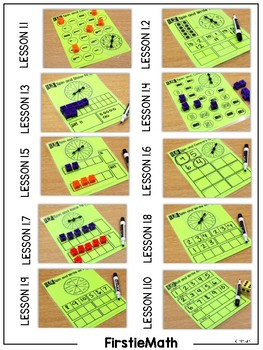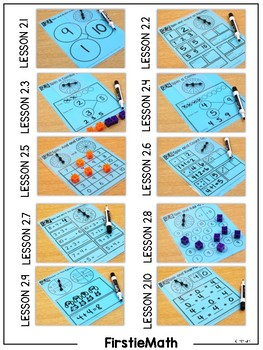# FirstieMath®: First Grade Math Aligned Center Mats | Homeschool Compatible |K - 1st, Homeschool
Subjects
Resource Type
Formats Included
• PDF
Pages
311 pages

### Description

FirstieMath®: Aligned Center Mats- A set of 300 differentiated centers that are easy to prep and highly engaging for students. This packet is designed for the first grade classroom and easily adapted to fit a guardian-led homeschool setting! If inquiring about this bundle for a homeschool setting, feel free to email me directly with any questions at tara@littlemindsatwork.org!

Do you have a few extra moments? LISTEN and WATCH more about FirstieMath: Aligned Center Mats HERE!

FirstieMath: Aligned Center Mats

FirstieMath Center Mats are an aligned supplement to the FirstieMath Curriculum program. However, the FirstieMath Aligned Center Mats can be paired with any first grade math curriculum successfully. The packet covers 15 units and includes 150 days of hands-on centers. Each center is offered in a differentiated approach so there is a total of 300 centers. There is a reinforce and expand version for each center. This will allow you to utilize the centers for differentiating your lessons. The centers are extremely easy to prep. You will simply print the center mats and place them into dry erase pouches, sheet protectors or laminate. The centers take NO color ink and require NO cutting. The students will simply need a copy of the task printed out and simple classroom manipulatives. The activities can be used during your whole-group math lesson as the follow-up task, intervention block, or for independent practice during a small-group math lesson rotation. Be sure to view the scope and sequence and the picture shots to see it in action!

What is included for FirstieMath: Aligned Center Mats?

- 300 center mats (150 for students needing a reinforce option and 150 additional center mats for students that are able to expand)

- Unit Labels

What units are covered for the FirstieMath: Aligned Center Mats?

Unit One: Numbers to 10 INCLUDED

Unit Two: Addition to 10 INCLUDED

Unit Three: Subtraction to 10 INCLUDED

Unit Four: Geometry INCLUDED

Unit Five: Numbers to 20 INCLUDED

Unit Six: Add and Subtract to 20 INCLUDED

Unit Seven: Measurable Attributes INCLUDED

Unit Eight: Graphing INCLUDED

Unit Nine: Numbers to 40 INCLUDED

Unit Ten: Add and Subtract to 40 INCLUDED

Unit Eleven: Math Strategies INCLUDED

Unit Twelve:Numbers to 120 INCLUDED

Unit Thirteen: Add and Subtract to 100 INCLUDED

Unit Fourteen: Time and Money INCLUDED

Unit Fifteen: 2nd Grade Prep INCLUDED

What skills are covered for the FirstieMath: Aligned Center Mats?

Lesson 1.1 Numbers 1-10

Lesson 1.2 Numbers 1-10

Lesson 1.4 Counting Numbers 1-10

Lesson 1.5 Comparing Numbers 1-5

Lesson 1.6 Comparing Numbers 6-10

Lesson 1.7 Comparing Numbers 1-10

Lesson 1.8 Number Patterns 1-5

Lesson 1.9 Number Patterns 6-10

Lesson 1.10 Number Patterns 1-10

Lesson 2.1 Number Bonds

Lesson 2.3 How to Make 4, 5, 6

Lesson 2.4 How to Make 7, 8, 9

Lesson 2.5 How to Make Numbers 1-10

Lesson 2.6 Counting on to Add

Lesson 2.7 Find the Missing Numbers

Lesson 2.10 Rearranging the Problem

Lesson 3.2 Subtract by Taking Away

Lesson 3.3 Subtract by Taking Away 2

Lesson 3.4 Subtract by Counting On

Lesson 3.5 Subtract by Counting Back

Lesson 3.6 Real World Subtraction Problems

Lesson 3.7 Rearranging Subtraction Problems

Lesson 3.8 Recognizing Fact Families

Lesson 3.9 Making Fact Families

Lesson 3.10 True/False Subtraction

Lesson 4.1 Plane Shapes: Circle and Square

Lesson 4.2 Plane Shapes: Rectangle and Triangle

Lesson 4.3 Plane Shapes: Alike and Different

Lesson 4.4 Equal Parts of a Shape

Lesson 4.5 3D Shapes: Sphere, Rect. Prism, Cone

Lesson 4.6 3D Shapes: Cube, Pyramid, Cylinder

Lesson 4.7 Building with 3D Shapes

Lesson 4.8 Real World Shapes

Lesson 4.9 Order of Shapes

Lesson 4.10 Patterns with Plane/3D Shapes

Lesson 5.1 Make 10 and Count On

Lesson 5.2 Place Value

Lesson 5.3 Place Value Continued

Lesson 5.4 Tens Frame Number to 20

Lesson 5.5 Exploring 20

Lesson 5.6 Compare Numbers 11-15

Lesson 5.7 Compare Numbers 16-20

Lesson 5.8 1-20 Number Order

Lesson 5.9 Number Patterns: More or Less

Lesson 5.10 Write Numbers 11-20

Lesson 6.2 Addition Doubles Plus 1

Lesson 6.3 Make a Ten to Add

Lesson 6.4 Add by Counting On

Lesson 6.5 Real World Addition Problems

Lesson 6.6 Subtraction Without Regrouping

Lesson 6.7 Subtraction With Regrouping

Lesson 6.8 Subtraction With Regrouping

Lesson 6.9 Real World Subtraction Problems

Lesson 6.10 Fact Families

Lesson 7.1 Comparing Length of 2 Objects

Lesson 7.2 Comparing Length of 3+ Objects

Lesson 7.3 Nonstandard Measurement

Lesson 7.4 Nonstandard Measurement

Lesson 7.5 Measuring in Units

Lesson 7.7 Comparing Weight of 2 Objects

Lesson 7.8 Comparing Weight of 3+ Objects

Lesson 7.9 Nonstandard Weight Measurement

Lesson 7.10 Weighing in Units

Lesson 8.1 Sort and Organize

Lesson 8.2 Picture Graphs

Lesson 8.3 Picture Graphs

Lesson 8.4 Tally Charts

Lesson 8.5 Bar Graphs

Lesson 8.6 Bar Graphs

Lesson 8.7 Line Graphs

Lesson 8.8 Line Graphs

Lesson 8.9 Circle Graphs

Lesson 8.10 Analyzing Graphs

Lesson 9.1 Place Value

Lesson 9.2 Place Value

Lesson 9.3 Comparing 2-Digit Numbers

Lesson 9.4 Comparing 2-Digit Numbers w/ Symbols

Lesson 9.5 Ordering Numbers

Lesson 9.6 Ordering Numbers

Lesson 9.7 Patterning Numbers

Lesson 9.8 Ten More and Ten Less

Lesson 9.9 Ten More and Ten Less

Lesson 9.10 Estimating Groups of Ten

Lesson 10.1 Adding Number Without Regrouping

Lesson 10.4 Real World Addition Problems

Lesson 10.5 Subtraction Without Regrouping

Lesson 10.6 Subtraction With Regrouping

Lesson 10.7 Subtraction With Regrouping

Lesson 10.8 Real World Subtraction Problems

Lesson 11.1 Number Bonds For Mental Math

Lesson 11.2 Mentally Add 1-Digit Numbers

Lesson 11.3 Mentally Add 1-Digit Numbers to a 2 Digit Number

Lesson 11.4 Add a 2 Digit Number to a Ten

Lesson 11.5 Subtract 1-Digit Numbers Mentally

Lesson 11.6 Mentally Subtract 1-Digit Number from 2-Digit Number

Lesson 11.7 Mentally Subtract Tens From a 2-Digit Number

Lesson 11.8 Identifying Patterns

Lesson 11.9 Addition to 40 Review

Lesson 11.10 Subtraction to 40 Review

Lesson 12.1 Counting to 120

Lesson 12.2 Counting to 120

Lesson 12.3 Place Value

Lesson 12.4 Place Value

Lesson 12.5 Comparing Numbers to 120

Lesson 12.6 Ordering Numbers to 120

Lesson 12.7 Ordering Numbers to 120

Lesson 12.8 Numbers Patterns to 120

Lesson 12.9 Estimating Numbers to 120

Lesson 12.10 Estimating Numbers to 120

Lesson 13.5 Subtraction Without Regrouping

Lesson 13.6 Subtraction Without Regrouping

Lesson 13.7 Subtraction With Regrouping

Lesson 13.8 Subtraction With Regrouping

Lesson 13.9 Estimating Sums to 100

Lesson 13.10 Estimating Differences to 100

Lesson 14.1 Ordering Events

Lesson 14.2 Time to the Hour

Lesson 14.3 Time to the Half Hour

Lesson 14.4 Telling Time to the Hour and Half Hour

Lesson 14.5 Penny, Nickel, Dime

Lesson 14.6 Learning the Quarter

Lesson 14.7 Counting Money

Lesson 14.8 Counting Money

Lesson 14.10 Subtracting Money

Lesson 15.1 Equal Parts

Lesson 15.2 Fractions: One Half

Lesson 15.3 One Third

Lesson 15.4 One Fourth

Lesson 15.5 Non-Unit Fractions

Lesson 15.6 Multiplication Prep: Adding the Same #

Lesson 15.7 Finding the Number of Groups

Lesson 15.8 Finding the Number of Groups

Lesson 15.9 Measure to the Nearest Inch

Lesson 15.10 Measure to the Nearest Inch

What is FirstieMath?

FirstieMath is a complete and comprehensive math curriculum. You can view more about FirstieMath HERE.

Do you teach KinderMath? Snag the KinderMath Aligned Center Mats!

HERE!

Visit my blog Little Minds at Work

Total Pages
311 pages
N/A
Teaching Duration
1 Year
Report this Resource to TpT
Reported resources will be reviewed by our team. Report this resource to let us know if this resource violates TpT’s content guidelines.